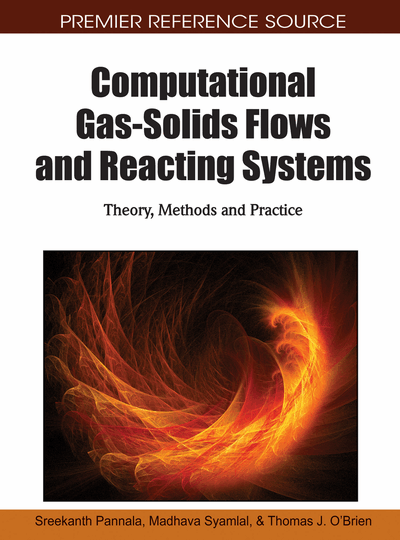# Quadrature-Based Moment Methods for Polydisperse Gas-Solids Flows

Alberto Passalacqua (Iowa State University, USA), Prakash Vedula (University of Oklahoma, USA) and Rodney O. Fox (Iowa State University, USA)
DOI: 10.4018/978-1-61520-651-3.ch007
Available
\$37.50
No Current Special Offers

## Abstract

Classical Euler-Euler two-fluid models based on the kinetic theory of granular flows assume the particle phase to be dominated by collisions, even when the particle volume fraction is low and hence collisions are negligible. This leads to erroneous predictions of the particle-phase flow patterns and to the inability of such models to capture phenomena like particle trajectory crossing for finite Stokes numbers. To correctly predict the behavior of dilute gas-particle flows a more fundamental approach based on solving the Boltzmann kinetic equation is necessary to treat non-zero Knudsen-number and finite Stokes-number conditions. In this chapter an Eulerian quadrature-based moment method for the direct solution of the Boltzmann equation is adopted to describe the particle phase, and it is fully coupled with an Eulerian fluid solver to account for the two-way coupling between the phases. The solution algorithm for the moment transport equations derived in the quadrature-based moment method and the coupling procedure with a fluid solver are illustrated. The predictive capabilities of the method are shown considering a lid-driven cavity flow with particles at finite Stokes and Knudsen numbers, and comparing the results with both Euler-Euler two-fluid model predictions and with Euler-Lagrange simulations.
Chapter Preview
Top

## Introduction

The quadrature method of moments (QMOM) was proposed for the first time to solve population balance equations by McGraw (1997) for studying size-dependent growth in aerosols, and then applied to aggregation problems by Barrett and Webb (1998). The method was extended by Wright et al. (2001) to treat bi-variate aerosol distributions. Marchisio et al. (2003a) tested the QMOM approach for solving the length-based population-balance equation for molecular growth and aggregation. Breakage processes were considered in Marchisio et al. (2003b), while the QMOM implementation in CFD codes was discussed in Marchisio et al. (2003c)

In the work of Marchisio (2003c), QMOM is applied to the solution of a population balance equation (PBE) in the size-based number density function, to treat aggregation and breakage phenomena. Fan et al. (2004) applied the direct quadrature method of moments (DQMOM) to study aggregation and breakage processes in gas-solid fluidized beds showing that the approach is very effective in modeling solid segregation and elutriation, and in tracking the evolution of the particle size distribution. Recently Strumendo & Arastoopour (2008) developed the Finite size domain Complete set of trial functions Method Of Moments (FC-MOM), where the size distribution function is represented as a series expansion by a complete system of orthonormal functions. The latter is useful when the functional shape of the number density function is needed. In comparison, QMOM provides only the moments but is computationally very efficient given its overall accuracy.

A particularly interesting use of QMOM is the solution of the Boltzmann kinetic equation, which represents the foundation of the kinetic theory of granular gases. Classical Euler-Euler multi-fluid models are based on conservation equations for the lower-order moments of the velocity-based number density function, the unknown in the Boltzmann equation. Closures for these equations are obtained under restrictive assumptions such as isotropy of the particle velocity fluctuations, and energy equipartition, assuming a Maxwellian velocity distribution. The latter hypothesis corresponds to considering collision-dominated flows, at the equilibrium condition, and imposes strong restrictions on the range of the Knudsen numbers, defined by the ratio of the mean free path and the characteristic length scale of the system (Jenkins & Savage, 1983; Gidaspow, 1994).

The behavior of a granular gas and its mathematical description are a function of its Knudsen number (Goldhirsch, 2005). In particular, in the limit of zero Knudsen number, an elastic granular gas can be described using the Euler equation, neglecting the viscous stresses in the Navier-Stokes equation. For Knudsen numbers between zero and 0.01, the Navier-Stokes equation with no-slip boundary conditions at walls provides a satisfactory description of the flow, while for Knudsen numbers between 0.01 and 0.1, partial slip boundary conditions are required to deal with the presence of a non-negligible Knudsen layer at the walls, where the rarefaction effects start to appear. For Knudsen numbers higher than 0.1, the Knudsen layer extends into the bulk of the granular gas, and the validity of the Navier-Stokes equation is questionable in the whole domain. In these conditions, higher-order terms in the Chapman-Enskog expansion or direct solutions of the Boltzmann equation are necessary to correctly describe the flow behavior (Bird, 1994, Goldhirsch et al., 2005, Galvin et al., 2007).

## Complete Chapter List

Search this Book:
Reset